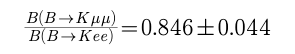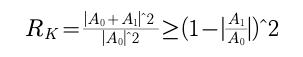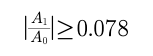# Can Higgs explain Leption Universality Violation in B->Kll ?

A few days ago, the LHCb collaboration reported an evidence for Lepton Universality Violation (LUV) in the beauty-quark decays B -> K l+ l- where l represents muon or electron. They measured the ratio of muon to electron to be (more details see their paper)This ratio is denoted by RK. The Standard Model (SM) in particle physics predicts 1.00+/-0.01. So this establishes the first evidence for the Lepton Universality Violation.

SM treats all electrons (eletron, muon and tau) equally except for Higgs. Higgs couples to fermions with a strength proportional to fermion mass. So in principle, Higgs might make such an asymmetry.The left diagram shows the dominant electro-weak contribution in SM while the right one shows a diagram with Higgs involved. The total contribution will be their vectorial sum. Let us have a rough estimation to see whether Higgs explains this LUV or not.Here A0 and A1 represent the amplitude in the left and right diagram, respectively. For RK=0.846, we findIs this number crazy? In SM, we havewhere I missed the electromagnetic coupling constant alpha=1/137 in numerator right after the second equal sign, but I don't want to modify it due to reasons you know; mc, mmu and m are the mass of charm quark, muon and Higgs; v is the vaccum expectation value; q2 is the momentum transfer (1-6 GeV2 as in the paper).

So, Higgs canNOT explain to the Lepton Universality Violation observed in B -> K l+ l-.

https://blog.sciencenet.cn/blog-3401446-1278485.html

## 全部精选博文导读

GMT+8, 2023-9-30 00:47